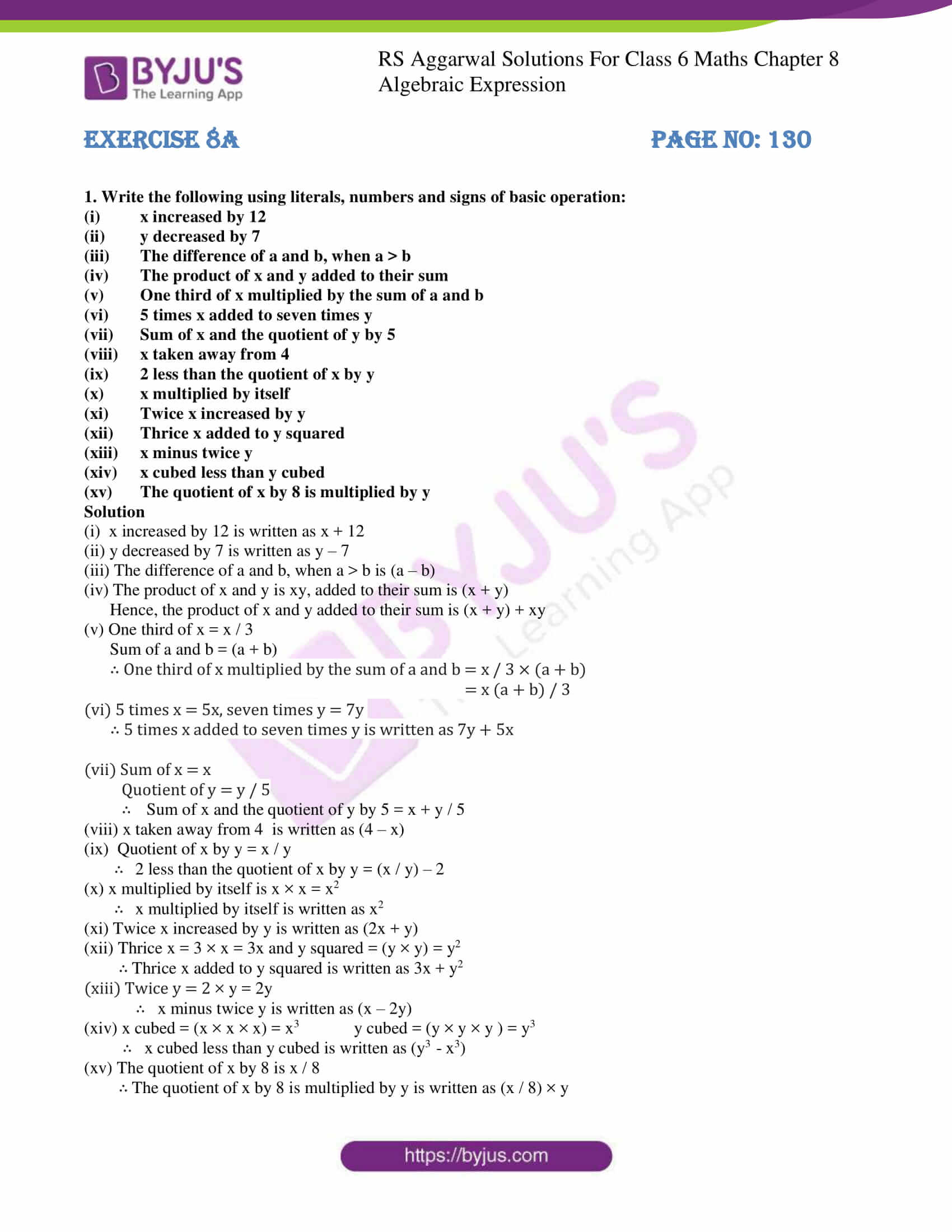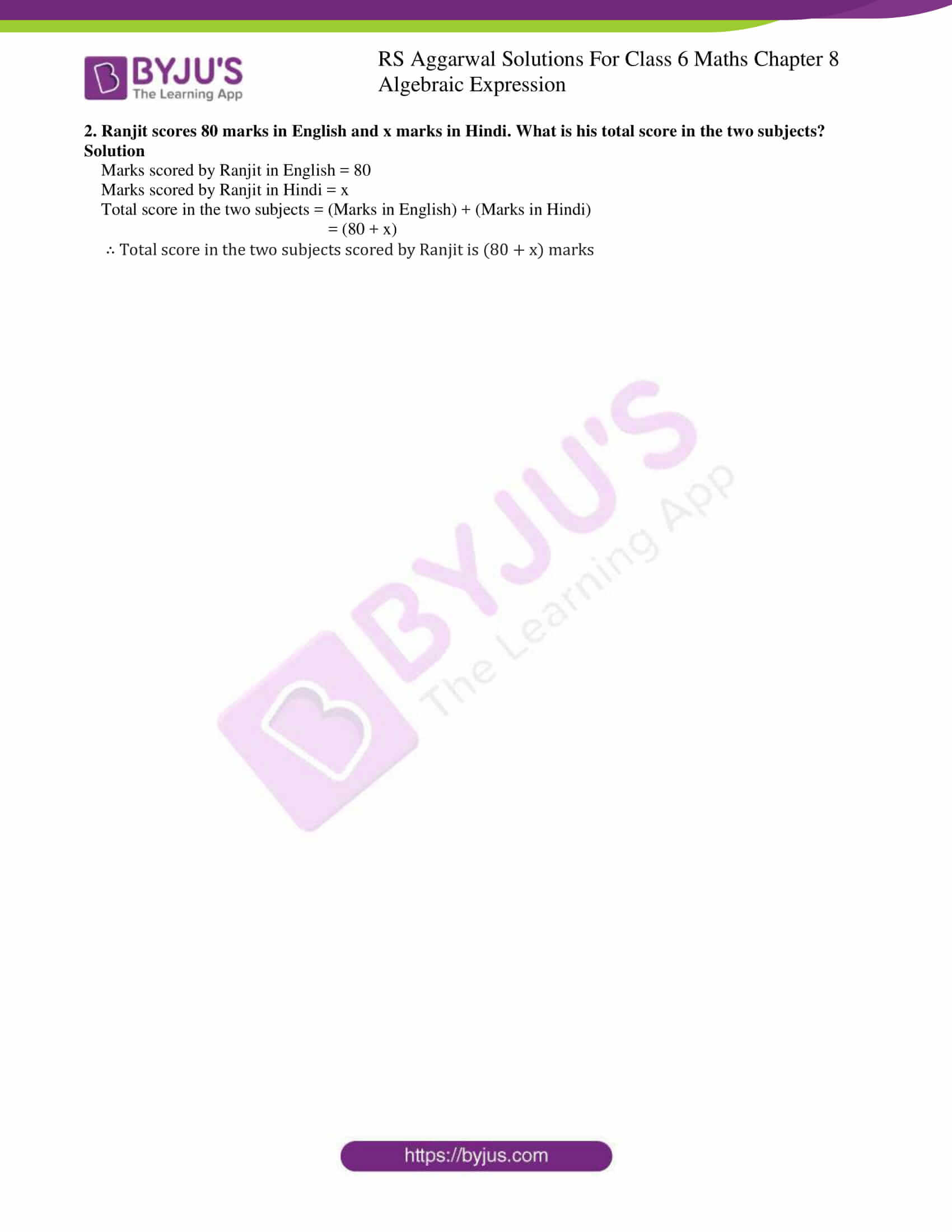# RS Aggarwal Solutions for Class 6 Chapter 8 Algebraic Expressions Exercise 8A

RS Aggarwal Solutions for Class 6 Chapter 8 Algebraic Expressions are provided below for students with the free download link. The first exercise of chapter 8 has questions on the concept of operations on literals and numbers. The faculty at BYJU’S have solved the textbook problems to help students with their exam preparation. Students who practice problems using solutions PDF obtain good marks in the exam and boost their confidence in solving problems.

## Download PDF of RS Aggarwal Solutions for Class 6 Chapter 8 Algebraic Expressions Exercise 8A### Access answers to Maths RS Aggarwal Solutions for Class 6 Chapter 8 Algebraic Expressions Exercise 8A

1. Write the following using literals, numbers and signs of basic operation:

(i) x increased by 12

(ii) y decreased by 7

(iii) The difference of a and b, when a > b

(iv) The product of x and y added to their sum

(v) One third of x multiplied by the sum of a and b

(vi) 5 times x added to seven times y

(vii) Sum of x and the quotient of y by 5

(viii) x taken away from 4

(ix) 2 less than the quotient of x by y

(x) x multiplied by itself

(xi) Twice x increased by y

(xii) Thrice x added to y squared

(xiii) x minus twice y

(xiv) x cubed less than y cubed

(xv) The quotient of x by 8 is multiplied by y

Solution

(i) x increased by 12 is written as x + 12

(ii) y decreased by 7 is written as y – 7

(iii) The difference of a and b, when a > b is (a – b)

(iv) The product of x and y is xy, added to their sum is (x + y)

Hence, the product of x and y added to their sum is (x + y) + xy

(v) One third of x = x / 3

Sum of a and b = (a + b)

∴ One third of x multiplied by the sum of a and b = x / 3 × (a + b)

= x (a + b) / 3

(vi) 5 times x = 5x, seven times y = 7y

∴ 5 times x added to seven times y is written as 7y + 5x

(vii) Sum of x = x

Quotient of y = y / 5

∴ Sum of x and the quotient of y by 5 = x + y / 5

(viii) x taken away from 4 is written as (4 – x)

(ix) Quotient of x by y = x / y

∴ 2 less than the quotient of x by y = (x / y) – 2

(x) x multiplied by itself is x × x = x2

∴ x multiplied by itself is written as x2

(xi) Twice x increased by y is written as (2x + y)

(xii) Thrice x = 3 × x = 3x and y squared = (y × y) = y2

∴ Thrice x added to y squared is written as 3x + y2

(xiii) Twice y = 2 × y = 2y

∴ x minus twice y is written as (x – 2y)

(xiv) x cubed = (x × x × x) = x3 y cubed = (y × y × y ) = y3

∴ x cubed less than y cubed is written as (y3 – x3)

(xv) The quotient of x by 8 is x / 8

∴ The quotient of x by 8 is multiplied by y is written as (x / 8) × y

2. Ranjit scores 80 marks in English and x marks in Hindi. What is his total score in the two subjects?

Solution

Marks scored by Ranjit in English = 80

Marks scored by Ranjit in Hindi = x

Total score in the two subjects = (Marks in English) + (Marks in Hindi)

= (80 + x)

∴ Total score in the two subjects scored by Ranjit is (80 + x) marks